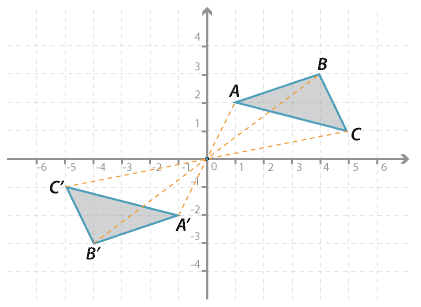### Rotation of 180 ° about the originDetailed description

In the diagram above, triangle $$ABC$$ is rotated 180 ° about the origin:

$$A(1, 2) \rightarrow A^\prime (−1, −2); B(4, 3) \rightarrow B^\prime (−4, −3); C(5, 1) \rightarrow C^\prime (−5, −1)$$.

### Combinations of rotations of 90 °

It is easy to see that two separate rotations of 90 ° in a clockwise direction is the same as rotating by 180 °.